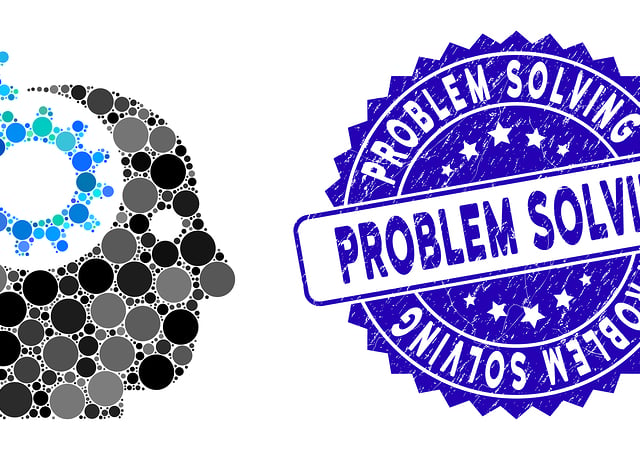# Formulas You’ll Need for the GMAT™ Quantitative Section’s Statistics and Problem Solving Questions

When you’re solving problems in the GMAT™ Quantitative Section, you need to be both fast and accurate. You cannot choose just one. If you want to ace the test, you’ll need to be able to understand the problem quickly, figure out the process to solve it, and actually perform the necessary calculations in less than 2 minutes. There are 31 questions in all and just 61 minutes to solve them.

To achieve that, you need to have the equations you need ingrained in your brain, and we have the resources you need. In the following chart, you’ll find the essential equations for the GMAT™ Quantitative Section Statistics and Problem Solving Questions. You’ll find formulas relating to the other types of questions on this section of the test here:

Arithmetic and Geometry

Algebra

Use them to solve our free sample problems.

For more in-depth information, check out our free study guide

## Statistics and Problem-Solving

Category Formula Symbols Comment
Statistics and
Problem
Solving
$$d=r \cdot t$$
$$w=r \cdot t$$
$$W=r \cdot t$$
d = distance
w = wage
W = Work done
r = rate
t = time

Statistics and
Problem
Solving
$$TW=WA+WB$$ TW = Total work done
WA = Work done by A
WB = Work done by B
Combined Work
Statistics and
Problem
Solving
$$\dfrac{1}{t} = \dfrac{1}{tA} + \dfrac{1}{tB}$$ t = total time
tA = time consumed by A
tB = time consumed by B
Combined Work
Statistics and
Problem
Solving
$$Du=Su \cdot \dfrac{Du}{Su}=Su \cdot CF$$ Du = Desired Unit
Su = Starting Unit
CF = Conversion Factor
Multiple steps may
be needed.
Statistics and
Problem
Solving
$$a \cdot b\% =a \cdot \frac{b}{100}$$ a = any real number
b% = any percent
Remember to simplify
if necessary
Statistics and
Problem
Solving
$$\% = \frac{\vert b-a \vert }{b} \cdot 100= \frac{c}{b} \cdot 100$$ % = % increase or decrease
a = new value
b = original value
c = amount of change

Statistics and
Problem
Solving
$$\overline{x}= \dfrac{\Sigma x_i}{n}$$ $$\overline{x}$$ = mean
$$x_i$$ = value of each measurement
n = number of measurements

Statistics and
Problem
Solving
$$Md=(\dfrac{n+1}{2})^{th} term$$ Md = median
n = number of measurements (odd)

Statistics and
Problem
Solving
$$Md=\dfrac{(\frac{n}{2})^{th} term + (\frac{n+1}{2})^{th} term }{2}$$ Md = median
n = number of measurements (even)

Statistics and
Problem
Solving
$$s=\sqrt{\Sigma(x_i-\overline{x})^2/(n-1)}$$ s = standard deviation
$$\overline{x}$$ = mean
$$x_i$$ = value of each measurement
n = number of measurements

Statistics and
Problem
Solving
$$V=s^2$$ v = Variance
s = standard deviation

Statistics and
Problem
Solving
$$CV=RSD=100 \cdot \dfrac{s}{\overline{x}}$$ CV = Coefficient of variation
RSD = Relative standard deviation
s = standard deviation

Statistics and
Problem
Solving
$$P(n, r)=\dfrac{n!}{(n-r)!}$$ P = number of permutations
n = total number of objects
r = number of objects to be chosen

Statistics and
Problem
Solving
$$C(n, r) = \dfrac{n!}{r! \cdot (n-r)!}$$ C = number of combinations
n = total number of objects
r = number of objects to be chosen

Statistics and
Problem
Solving
$$p = \dfrac{d}{t}$$ p = probability of an event
d = number of ways desired event
can occur
t = total number of possible events

Statistics and
Problem
Solving
$$x_n = x_1 + d(n-1)$$ $$x_n = nth$$ element of an arith. sequence
$$x_1 = 1st$$ element of an arith. sequence
d = common difference
Arithmetic Sequence
Statistics and
Problem
Solving
$$x_n = x_1 \cdot r^{(n-1)}$$ $$x_n = nth$$ element of a geom. sequence
$$x_1 =$$ first element of a geom. sequence
d = common difference
Geometric Sequence
Statistics and
Problem
Solving
$$SI = P \cdot IR \cdot t$$ SI = Simple Interest
P = Principal (amount borrowed)
IR = Interest Rate
t = time (in same units as IR)

Statistics and
Problem
Solving
$$A_{SI} = P + SI = P \cdot (1+(IR \cdot t))$$ $$A_{SI}$$ = Future value to be paid
(for SI)
P = Principal (amount borrowed)
SI = Simple Interest
IR = Interest Rate
t = time (in same units as IR)

Statistics and
Problem
Solving
$$A_{CI} = P \cdot (1 + \frac{IR}{n})^{n \cdot t}$$ $$A_{CI}$$ = Future value to be paid
(for CI)
P = Principal (amount borrowed)
IR = Interest Rate
n = Number of times interest is compounded
per unit of time
t = time (in same units as IR)